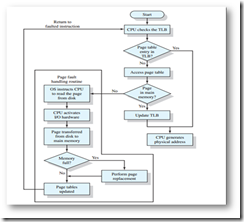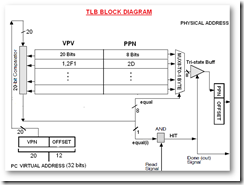## Thursday, June 7, 2012

### Simple Memory Management Unit with VHDL

I had an assignment to design a simple memory management unit that takes virtual address, looks up this address in TLB (translation lookaside buffer), if found in the TLB, read the physical address from it and adds the offset. if not found in TLB, then looks into the page table inside the memory.
Block diagram for this systemI have implemented all except the page fault handling routine.
The TLB design is simply a 8 rows of 28 bits register, the first 20 bits contain the virtual page number,  connected to a comparator, the comparator is also connected to the input page number. the comparator output is connected to tri-state buffer control pin. the input to the tri-state buffers is the last 8 bits of the register that represents the physical address. When the comparator output is “1”, this passes the physical address through the tri-state buffer.
below is a block diagram for the TLBMMU entities
Package used to define the input
```
library ieee; use ieee.std_logic_1164.all;
package byte_pkg is
subtype byte is std_logic_vector(7 downto 0);
type byte_array_t is array(natural range <>) of byte;
end;
```

tri-state buffer
```
library IEEE;
use IEEE.STD_LOGIC_1164.ALL;

entity tbuff is
PORT(
inp:IN STD_LOGIC_VECTOR(7 DOWNTO 0);
sel:IN STD_LOGIC;
outp:OUT STD_LOGIC_VECTOR(7 DOWNTO 0));
end tbuff;

architecture Behavioral of tbuff is
begin
outp <= "ZZZZZZZZ"
WHEN (sel = '0')
ELSE inp;
end Behavioral;
```

comparator
```
library IEEE;
use IEEE.STD_LOGIC_1164.all;

entity comparator is
port(
IN1:in std_logic_vector(19 downto 0);
IN2:in std_logic_vector(19 downto 0);
equal:out std_logic);
end comparator;

architecture Behavioral of comparator is
begin
process(IN1, IN2)
begin

if (IN1 = IN2 ) then
equal <= '1';
else
equal <= '0';
end if;
end process;
end Behavioral;
```

TLB output multiplexer
```
library IEEE;
use IEEE.STD_LOGIC_1164.ALL;
use work.byte_pkg.all;

entity out_sel is
Port( inp:in byte_array_t(0 to 7);
outp:out std_logic_vector(7 downto 0);
selector:in std_logic_vector(7 downto 0));
end out_sel;

architecture Behavioral of out_sel is
begin
process(selector,inp)
begin
CASE selector IS
WHEN"00000001"=>outp<=inp(0);
WHEN"00000010"=>outp<=inp(1);
WHEN"00000100"=>outp<=inp(2);
WHEN"00001000"=>outp<=inp(3);
WHEN"00010000"=>outp<=inp(4);
WHEN"00100000"=>outp<=inp(5);
WHEN"01000000"=>outp<=inp(6);
WHEN"10000000"=>outp<=inp(7);
WHEN OTHERS=>
outp<="ZZZZZZZZ";
END CASE;
end process;

end Behavioral;

```

TLB – uses generate statement to create 8 comparators, tri-state buffers

```
library ieee;
use ieee.std_logic_1164.all;
use ieee.std_logic_arith.all;
use work.byte_pkg.all;

entity TLB is
port (clock: in std_logic;
pn:in std_logic_vector(19 downto 0);
fn_in:in std_logic_vector(7 downto 0);
fn_out:out std_logic_vector(7 downto 0);
r:in std_logic;
w:in std_logic;
hit:out std_logic);
end TLB;
architecture Behavioral of TLB is
component tbuff
PORT( inp:IN std_logic_vector(7 DOWNTO 0);
sel:IN std_logic;
outp:OUT std_logic_vector(7 DOWNTO 0));
end component;
component comparator
Port(
IN1:in std_logic_vector(19 downto 0);
IN2:in std_logic_vector(19 downto 0);
equal:out std_logic);
end component;
component out_sel
Port( inp:in byte_array_t(0 to 7);
outp:out std_logic_vector(7 downto 0);
selector:in std_logic_vector(7 downto 0));
end component;
type tlb_type is array (0 to 7) of
std_logic_vector(31 downto 0);
signal tlb: tlb_type;--type eqtype is array (0 to 7) of std_logic;
signal equal:std_logic_vector(7 downto 0);
signal out_array:byte_array_t(0 to 7);
signal Counter: integer range 0 to 7;
signal tempfn:std_logic_vector(7 downto 0);
begin
-- initializing the 8 comparators, tri-state buffers
comps:for i in 0 to 7 generate
compx: comparator port map(tlb(i)(27 downto 8),pn , equal(i));
tbuffx: tbuff port map(tlb(i)(7 downto 0), equal(i) , out_array(i));
end generate comps;
--inializing the output multiplexer
MUX:out_sel port map (out_array, tempfn, equal);

process (clock)
begin
if (clock'event and clock='1') AND r='1' then
if equal(0) = '1' or equal(1) = '1' or equal(2) = '1' or equal(3) = '1' or equal(4) = '1'or equal(5) = '1' or equal(6) = '1' or equal(7) = '1' then
hit<='1';
fn_out<=tempfn;
else
hit <='0';
fn_out <= (others => '0');
end if;
end if;
end process;
process (w, clock)
begin
if (clock'event and clock='1')  AND w='1' then
tlb(Counter) <= "0001" & pn & fn_in;
if Counter < 7 then
Counter <= Counter +1;
else
Counter <=0;
end if;
end if;
end process;
end Behavioral;
```

RAM
```
library IEEE;
use IEEE.STD_LOGIC_1164.ALL;
use IEEE.STD_LOGIC_ARITH.ALL;
use IEEE.STD_LOGIC_UNSIGNED.ALL;

entity testram is Port (
data:     out std_logic_vector(7 downto 0)
);
end testram;

architecture behavioral of testram is

type mem_array is array (0 to 79) of std_logic_vector(7 downto 0);
constant characters: mem_array := (

"00000001",
"00000010",
"00000011",
"00000100",
"00000101",
"00000110",
"00000111",
"00001000",
"00001001",
"00001010",
"00001011",
"00001100",
"00001101",
"00001110",
"00001111",
"00010000",
"00010001",
"00010010",
"00010011",
"00010100",
"00010101",
"00010110",
"00010111",
"00011000",
"00011001",
"00011010",
"00011011",
"00011100",
"00011101",
"00011110",
"00011111",
"00100000",
"00100001",
"00100010",
"00100011",
"00100100",
"00100101",
"00100110",
"00100111",
"00101000",
"00101001",
"00101010",
"00101011",
"00101100",
"00101101",
"00101110",
"00101111",
"00110000",
"00110001",
"00110010",
"00110011",
"00110100",
"00110101",
"00110110",
"00110111",
"00111000",
"00111001",
"00111010",
"00111011",
"00111100",
"00111101",
"00111110",
"00111111",
"01000000",
"01000001",
"01000010",
"01000011",
"01000100",
"01000101",
"01000110",
"01000111",
"01001000",
"01001001",
"01001010",
"01001011",
"01001100",
"01001101",
"01001110",
"01001111",
"01010000"
);
begin

begin
end process;

end behavioral;
```

MMU – State machine
```
library IEEE;
use IEEE.STD_LOGIC_1164.ALL;
entity MMU is
Port(clock:in std_logic;
ram_data:in std_logic_vector(7 downto 0);
enable:in std_logic;
r:in std_logic);
end MMU;
architecture Behavioral of MMU is
component TLB is
port (clock: in std_logic;
pn:in std_logic_vector(19 downto 0);
fn_in:in std_logic_vector(7 downto 0);
fn_out:out std_logic_vector(7 downto 0);
r:in std_logic;
w:in std_logic;
hit:out std_logic);
end component;

signal tlb_pageno: std_logic_vector(19 downto 0);
signal tlb_framenoin: std_logic_vector(7 downto 0);
signal tlb_framenoout: std_logic_vector(7 downto 0);
signal tlb_r: std_logic:= '0';
signal tlb_w: std_logic:= '0';
signal tlb_hit:std_logic:='Z';

TYPE STATE_TYPE IS (s0, s1, s2, s3, s4, s5, s6);
SIGNAL state   : STATE_TYPE;

begin
TLB1:TLB port map (clock, tlb_pageno, tlb_framenoin, tlb_framenoout, tlb_r, tlb_w,tlb_hit);
process(clock, enable, r)
begin
IF enable = '0' THEN
state <= s0;
tlb_r <= '0';
tlb_w <= '0';
ELSIF (clock'EVENT AND clock = '1') THEN
CASE state IS
WHEN S0=>
tlb_r <= '0';
tlb_w <= '0';
if r = '1' then
state <= s1;
end if;
WHEN s1=>
tlb_r<='1';
tlb_w<='Z';
state <= s2;
WHEN s2=>
tlb_r<='0';
IF tlb_hit = '1' THEN
state <= s4;
ELSIF tlb_hit = '0' then
state <= s3;
END IF;
WHEN s3=>
state <= s5;
WHEN s4=>
state <= s0;
WHEN s5=>
tlb_framenoin <= ram_data;
tlb_w <= '1';
state <= s0;
when others =>
state <= s0;
END CASE;
END IF;
END PROCESS;
end Behavioral;
```

Full circuit
```
library IEEE;
use IEEE.STD_LOGIC_1164.ALL;

entity crct is
Port(clock:in std_logic;
enable:in std_logic;
r:in std_logic);
end crct;

architecture Behavioral of crct is
component MMU is
Port(clock:in std_logic;
ram_data:in std_logic_vector(7 downto 0);
enable:in std_logic;
r:in std_logic);
end component;
component testram is
Port (
data:     out std_logic_vector(7 downto 0)
);
end component;
signal ram_data:std_logic_vector(7 downto 0);
begin
end Behavioral;
```

Test bench
```
LIBRARY ieee;
USE ieee.std_logic_1164.ALL;
USE ieee.numeric_std.ALL;
ENTITY TB_full IS
END TB_full;
ARCHITECTURE behavior OF TB_full IS
COMPONENT crct
Port(clock:in std_logic;
enable:in std_logic;
r:in std_logic);
END COMPONENT;

signal clock : std_logic := '0';
signal address_in : std_logic_vector(31 downto 0) := (others => '0');
signal r:std_logic:='0';
signal enable:std_logic:='0';
signal address_out : std_logic_vector(19 downto 0);
constant clock_period : time := 10 ns;

BEGIN
uut: crct PORT MAP (
clock => clock,
enable => enable,
r => r);
clock_process :process
begin
clock <= '0';
wait for clock_period/2;
clock <= '1';
wait for clock_period/2;
end process;
stim_proc: process
begin
wait for 100 ns;

wait for clock_period*10;
enable <= '1';
r<='1';